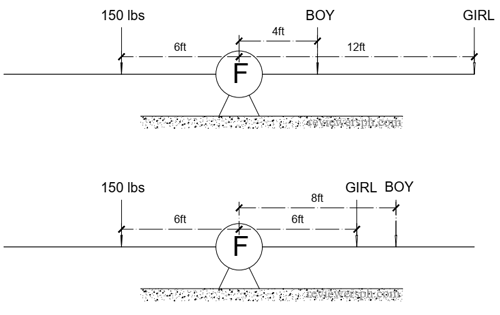### Math Notes

Subjects

#### Algebra Solutions

##### Topics || Problems

When we placed a boy 4 feet from the fulcrum, and a girl 12 feet from the fulcrum on one side of a teeterboard, they balanced a man weighing 150 pounds who is seated 6 feet from the fulcrum on the other side. Also, the board is balanced if the boy moves to 8 feet and the girl to 6 feet from the fulcrum on their side. Find their weight. Solution:Since the weight is balanced on both sides, this implies that the moment at the fulcrum is zero. It simply means that, the summation of the product of the weight the distance on one side is the same to the other side of the teeterboard.

$$6(150) = Boy (4) +12 (Girl)$$

$$6(150) = Boy (8) +6 (Girl)$$

$$Boy = -3 (Girl) + 225$$

$$900 = 8(-3Girl+ 225) +6 (Girl)$$

$$-900+1800= 30 (Girl)$$

$$Girl = 50$$

$$Boy = -3 (50) + 225 = 75$$

Thus the weight of the boy is 75 lbs and the girl is 50 lbs.